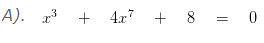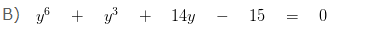# Polynomial in Hindi | Degree of a Polynomial Example

Polynomial in Hindi, deals with various concepts which are as under:-

• Polynomial (बहुपद )
• Coefficient ( गुणांक )
• Degree of the Polynomial
• Zero Polynomial  (शून्य बहुपद )
• Constant Polynomial (अचर बहुपद )
• Linear Polynomial  (रैखिक बहुपद)
• Cubic Polynomial  (त्रघाती बहुपद)
• Value of a Polynomial (बहुपद का मान )
• Zero of the Polynomial  (बहुपद के शून्यंक)
• Degree of a Polynomial Example

#### Polynomial (बहुपद )

A polynomial is an algebraic expression of the form p(x) = an xn + an-1 xn-1 +……..+ a1 x1 + a0, where an, an-1, a1, a0 are real numbers and an≠ 0 is called a polynomial.

where an xn , an-1 xn-1 +…….., a1 x1 , a0, are the terms of a polynomial and an , an-1 ,…….., a1, a0 are their coefficients.

#### Coefficient ( गुणांक )

The real number that precedes the variable is called the coefficient.

A polynomial involving one variable is called a polynomial in one variable.

#### Degree of the Polynomial

The exponent of the highest power of the variable of a polynomial is called the degree of the polynomial.

Based on its degree, a polynomial can be called as linear polynomial, quadratic polynomial, cubic polynomial and so on.

#### Zero Polynomial  (शून्य बहुपद )

The polynomial with all the coefficients as zeros is called a zero polynomial.

#### Constant Polynomial (अचर बहुपद )

A polynomial with a single term of a real number is called a constant polynomial.

#### Linear Polynomial  (रैखिक बहुपद)

A polynomial of degree one is called a first-degree or linear polynomial. The general form of a linear polynomial is ax + b, where a and b are real numbers and a ≠ 0.

A polynomial of degree two is called a second degree or quadratic polynomial. The general form of a quadratic polynomial is ax2 + bx + c, where a,b and c are real numbers and a ≠ 0.

#### Cubic Polynomial  (त्रघाती बहुपद)

A polynomial of degree three is called a third-degree or cubic polynomial. The general form of a cubic polynomial is ax3 + bx2 + cx + d, where a,b,c and d are real numbers and a ≠ 0.

A polynomial of degree four is called a fourth-degree or biquadratic polynomial. The general form of a quadratic polynomial is ax4 + bx3 + cx2 + dx + e, where a,b,c,d and e are real numbers and a ≠ 0.

#### Value of a Polynomial (बहुपद का मान )

The value of a polynomial p(x) when x = k (k is a real number) is the value obtained by substituting x as k. It is denoted by p(k).

#### Zero of the Polynomial  (बहुपद के शून्यंक)

The zero of the polynomial is defined as any real value of x, for which the value of the polynomial becomes zero.

### Degree of a Polynomial Example

#### निम्न बहुपदों की घात पता कीजिए?In this polynomial the highest power of the variable is 7 so the degree of the polynomial will be 7.In this polynomial the highest power of the variable y is 6 so the degree of the polynomial will be 7.

C) 15

In this there are no variable so the degree will be 0. The polynomial with degree 0 is called as constant.